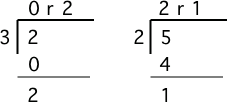# Expressions¶

The right hand side of the assignment statement doesn’t have to be a value. It can be an arithmetic expression, like `2*2`. The expression will be evaluated and the result from the expression will be stored in the variable.

##Teacher Note: Assignment Dyslexia¶

Some students will write the expression on the left side of the `=` and the variable name on the right side (`2 * 2 = a`). This is called assignment dyslexia and will result in an error. The variable name always has to be on the left side of the `=` in an assignment.

## Integer Division¶

You can use all the standard mathematical symbols.

Note

This book is using Python 3.0 which returns a decimal value from an integer calculation like `1 / 3`. If we had executed `1 / 3` in an older Python development environment it would have printed `0` instead. In many languages if you are only using integers in calculations (whole numbers - like -3,65, -39028, 602939) the result will also be an integer and the factional part (part after the decimal point) is thrown away. In those environments it is important to use decimal values (like `1.0 / 2`, `1 / 2.0`, or `1.0 / 2.0`) if you want a decimal result.

## Modulo¶

There are also some symbols that may be used in ways that you don’t expect.

You may not be familiar with the modulo (remainder) operator `%`. It returns the remainder when you divide the first number by the second. You probably did this long ago when you were learning long division. In the case of `4 % 2`, `2` goes into `4` two times with a remainder of `0`. The result of `5 % 2` would be `1` since `2` goes into `5`, two times with a remainder of `1`. In fact you can check if the result of `X % 2` is equal to `1` to see if `X` is odd and if the result of `X % 2` is equal to `0` then `X` is even.Figure 3: Long division showing the whole number result and the remainder¶

Note

The result of `x % y` when `x` is smaller than `y` is always `x`. The value `y` can’t go into `x` at all, since `x` is smaller than `y`, so the result is just `x`. So if you see `2 % 3` the result is `2`. Edit the code above to try this for yourself. Change the code to `result = 2 % 3` and see what that prints when it is run.